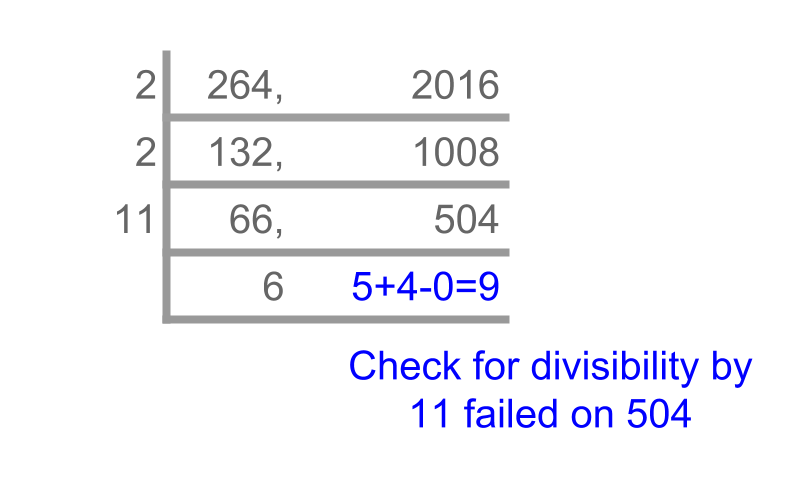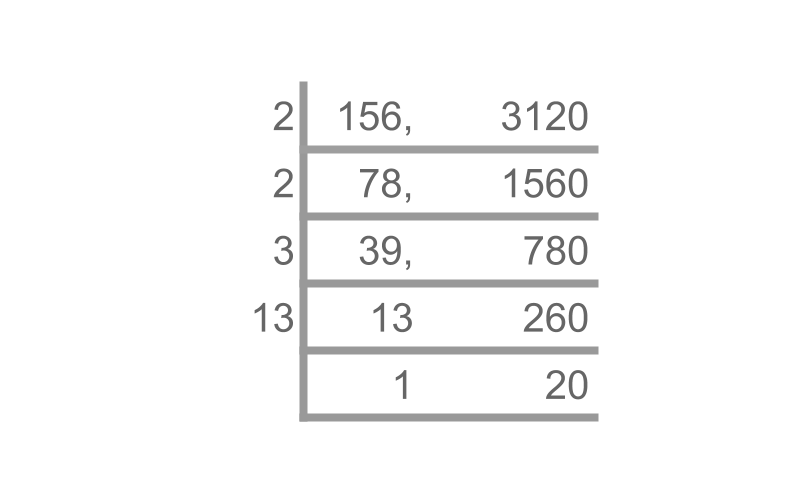maths > wholedivisors

Simplification of Divisibility Tests

what you'll learn...

overview

The following are explained

•  Simplification of divisibility test by subtraction

•  Simplification of divisibility test by division

•  Simplification of divisibility test by factors

These are used to develop other divisibility tests for specific numbers.

•  the divisibility test for 8$8$

•  the divisibility test for 12$12$

•  the divisibility test for 15$15$

Divisibility: Simplification by Subtraction

Consider the problem : Given a number 83×2322=192726$83 \times 2322 = 192726$. is it divisible by 83$83$?
The solution is simple. The number is given as a multiple of 83$83$, so it is divisible by $83$.

Consider another number $83 \times 23 + 422 = 2331$, Knowing that left addend $83 \times 23$ is divisible by $83$, we can check the smaller number $422$ for divisibility which is easier.
That is, checking $422$ for divisibility is good enough to conclude divisibility of the number $2331$

If only the number $2331$ is given, then it can be simplified to a smaller number $2331 - 83 \times 20 = 671$ which is easier to deal with for divisibility test.

To check the divisibility, the following properties are helpful.

•  multiple of a number is divisible by the number.
eg: $3 \times 423$ is a multiple of $3$ and so it is divisible by $3$.

•  In a sum of two numbers, if one number is a multiple of the divisor, then only the other number is checked for divisibility.
eg: Divisibility test of $3 \times 423 + 7$ by $3$, can be simplified to divisibility test of $7$, as $3 \times 423$ is a multiple of $3$.

•  To check divisibility of a large number by a divisor, it can be simplified into a smaller number by subtracting a multiple of the divisor.
eg: Divisibility test of $913$ by $3$, can be simplified to divisibility test of $913 - 300 \times 3 = 13$

The method is named Simplification by Subtraction method.

Simplification by Subtraction for Divisibility Tests: To perform divisibility test on a large number, a multiple of a divisor can be subtracted and the divisibility test is performed on the difference.

Find the divisibility of $777012$ by $111$.
Not Divisible. Subtract $777012 - 111 \times 7000 = 12$. Check the divisibility of $12$

Find the divisibility of $804$ by $67$.
Divisible. $134 = 67 \times 2$ is divisible by $67$

Divisibility: Simplification by Division

Given a number $340$. is it divisible by $21$?
$340 - 210 = 130$ and $130$ is not divisible by $21$. So, the number $340$ is not divisible by $21$

Consider divisibility of $340$ by $21$.

An observation is that the dividend is an even number and the divisor is not. The dividend can be written as $2 \times 170$.

The divisibility test can be performed on $170$ as the factor $2$ is a co-prime of $21$.
This can further be simplified as $170 \div 2 \div 5 = 17$, as $2$ and $5$ are co-primes of $21$.

To check the divisibility of a composite dividend by a divisor, the factors of the dividend can help in simplifying the divisibility test.

•  If a factor of dividend is co-prime of divisor, then the dividend can be divided by the co-prime factor and the divisibility test can be performed on the result.

This is named as Simplification by Division.

Simplification by Division: Dividend can be divided by a factor, that is co-prime of divisor, and the result is checked for divisibility.

Check divisibility of $990$ by $13$
$10$ and $9$ are co-primes of $13$. So the number is divided as $990 \div 90 = 11$. The result $11$ is not divisible by $13$. So $990$ is not divisible by $13$.

Divisibility: Simplification by Factors

Given a number $2321$. Is it divisible by $58$?
$2321 - 58 \times 4 = 1$. So, the number is not divisible by $58$

Consider the divisibility of dividend $2321$ by $58$.
An observation is that if a number is multiple of divisor $58$, then the number can be written as $58 \times n$. The same can be written as $2 \times 29 \times n$, because $58 = 2 \times 29$.

This implies that "the dividend is a multiple of $2$ and $29$". Since $2321$ is an odd number, it is not divisible by $2$ and so it is not divisible by $58$.

Consider the divisibility of $922$ by $58$.
It is observed that both are even numbers. So $922 = 2 \times 461$ and $58 = 2 \times 29$.

This implies that "the factor $461$ should be a multiple of $29$ for the divisibility test to pass". That is divisibility test of $922$ by $58$ is now changed to divisibility test of $461$ by $29$. The common factor $2$ is removed to simplify.

To check the divisibility of a dividend by a composite divisor, the factors of the divisors can help in simplifying the divisibility.

•  If the dividend is not divisible by a factor of divisor, then the dividend is not divisible by the composite divisor.
eg: divisibility of $2321$ by $58$. $58 = 2 \times 29$. The dividend $2321$ is not divisible by a factor of divisor $2$, so $2321$ is not divisible by $58$.

•  If the dividend is divisible by a factor, then both the dividend and divisor can be divided by the factor and the test is done on the results.
eg: Divisibility of $922$ by $58$. Both has common factor $2$ and so it is simplified as divisibility of $461$ by $29$. This is named as Simplification by Factors.

Simplification by Factors: To simplify divisibility test of dividend by a divisor,

•  any common factors can be removed from both the numbers and divisibility test can be performed on simplified dividend by the simplified divisor.

•  the dividend has to have all the factors of the divisor to pass the divisibility test.Check divisibility of $2016$ by $264$
The figure shows a simplified procedure by which simplification by factors can be performed.Check divisibility of $3120$ by $156$
The figure shows the simplified procedure by which simplification by factors is performed.

Divisibility by 8

The multiples of $8$ have the property that lowest $3$ digits (hundreds, tens and units) is divisible by $8$.

Explanation for the curious mind
A large number $2344$ can be given in the form $2 \times 1000 + 344$.
It is learned that if one addend is divisible, then the divisibility test can be performed only on the second addend.
$2 \times 1000$ is divisible by $8$ as $1000 = 125 \times 8$.
So the divisibility test is performed on $344$, that is the last $3$ digits of the number.

Test for Divisibility by $8$ : If the last three digits of the dividend is divisible by $8$, then the dividend is divisible by $8$.

Is $2018$ divisible by $8$?
Checking the last three digits $18$. As $18$ is not a multiple of $8$, it is concluded that the number is not divisible by $8$.

Is $7120$ divisible by $8$?
Checking the last three digits $120$. As $120$ is a multiple of $8$, it is concluded that the number is divisible by $8$.

Is $73524$ divisible by $8$?
Checking the last three digits $524$. The divisibility test can be simplified by subtraction ($524 - 400 - 80 - 40$) or simplified by factors ($\left(524 , 8\right)$ simplified to $\left(262 , 4\right)$, then to $\left(131 , 2\right)$).

Divisibility by 12

If a number is given as $3 \times 4 \times 2351$, will it be divisible by $12$?
It is divisible by $12$ as it has $3$ and $4$ as factors"

Test for Divisibility by $12$ : If a number is divisible by $3$ and $4$, then the number is divisible by $12$.

Is $2008$ divisible by $12$?
The number is divisible by $4$ and not divisible by $3$. So, it is concluded that the number is not divisible by $12$.

Is $720$ divisible by $12$?
The number is divisible by $4$ and $3$, So, it is concluded that the number is divisible by $12$.

Divisibility by $15$

Consider the number $3 \times 5 \times 2351$. It is divisible by $15$ as it has $3$ and $5$ as factors.

Test for Divisibility by $15$ : If a dividend is divisible by $3$ and divisible by $5$ then it is divisible by $15$

Is $2000$ divisible by $15$?
The number is divisible by $5$ but not by $3$. So it is not divisible by $15$.

Is $990$ divisible by $15$?
The number is divisible by $3$ and also divisible by $5$. So it is divisible by $15$.

summary

Simplification by Subtraction for Divisibility Tests: To perform divisibility test on a large number, a multiple of a divisor can be subtracted and the divisibility test is performed on the difference.

Simplification by Division: Dividend can be divided by a factor, that is co-prime of divisor, and the result is checked for divisibility.

Simplification by Factors: To simplify divisibility test of dividend by a divisor,

•  any common factors can be removed from both the numbers and divisibility test can be performed on simplified dividend by the simplified divisor.

•  the dividend has to have all the factors of the divisor to pass the divisibility test.

Test for Divisibility by $8$ : If the last three digits of the dividend is divisible by $8$, then the dividend is divisible by $8$.

Test for Divisibility by $12$ : If a number is divisible by $3$ and $4$, then the number is divisible by $12$.

Test for Divisibility by $15$ : If a dividend is divisible by $3$ and divisible by $5$ then it is divisible by $15$

Outline

The outline of material to learn "Divisibility in Whole Numbers" is as follows.

→   Classification as odd, even, prime, and composite

→   Factors, Multiples, Prime factorization

→   Highest Common Factor

→   Lowest Common Multiple

→   Introduction to divisibility tests

→   Simple Divisibility Tests

→   Simplification of Divisibility Tests

→   Simplification in Digits for Divisibility Tests